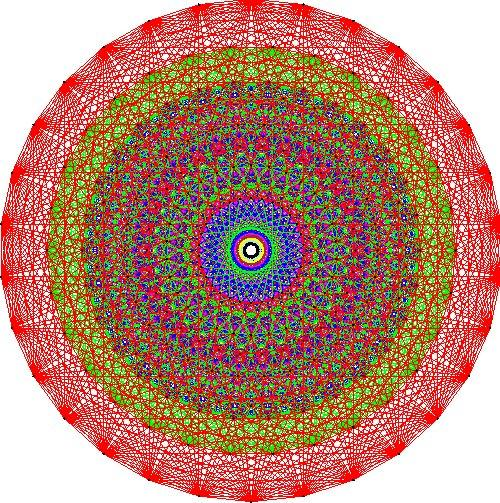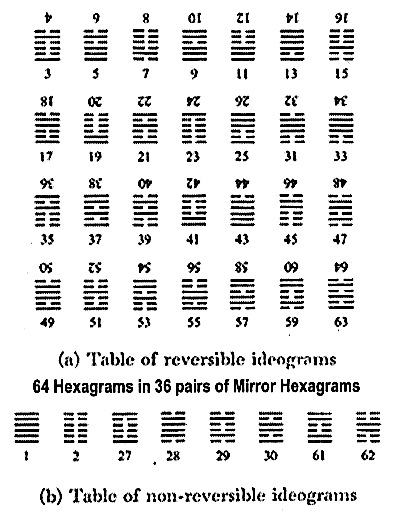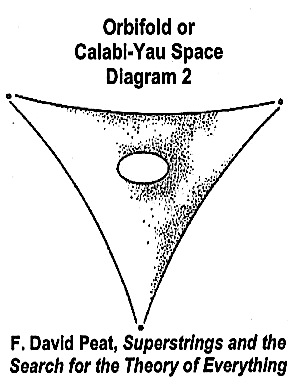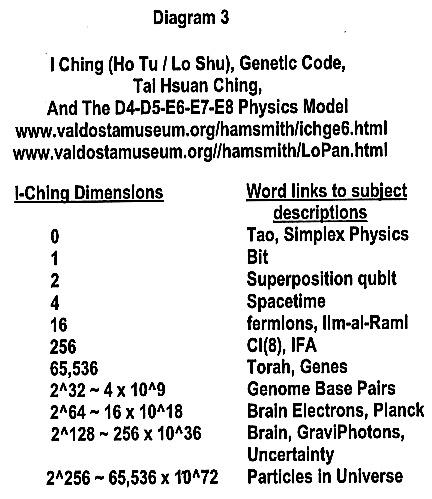## Mappings of Strings & Line Dimensions

Presented in this posting are the analogies between String Theories and I-Ching Logic within their respective dimensions. The dimensions of String Theories are computer-executing dimensions in the Information vacuum of hyperspace. On the other hand the I-Ching logic dimensions represent the pre-encoded Logic-Information potentials inside the participatory universe’s self-programmable computer. The former generates the dimension of the Information-Energy Vacuum, while the latter represents the pre-encoded Logic-Information Vacuum dimensions. The analogy between the two sets of dimensions will be illustrated.

Let us begin with these particular Vacuum Holons shown below.

a. The Vacuum in Numbers—Hyperspace—Mandalas

The above is one of the many Holons that define the vacuum in relationship to one’s left-right brain frame of references depending on the particular Observer. The following are two more.

b. The Vacuum —-> The Holon Logic of {[Void]—[Inside Zero]—[Nothing yet Everything]}

c. The Vacuum —-> Consciousness—Moment of Now—Teleportation of i

Now, let us examine the three components of Holon (a).

• Numbers —-> Base-9, Base-10, and so on
• Number —-> Base-9 + Void (The Logic Dimensions inside Zero or inside the Logic Vacuum) —-> 10 Dimensions

• Hyperspace —-> 10 dimensional Strings (Information-Energy Dimensions in the Information Vacuum)

STRINGS AND LINES: THE TEN DIMENSIONS IN I-CHING LOGIC

4 States: Mapping between Strings and Line Logic

• Strings <——> Lines:
• Open Strings <——> Yin Lines,
• Closed Strings <——> Yang Lines,
• Connecting Open and Closed Strings <——> A State in Neutral Lines
• No Connection <——> A State in Neutral Line

5 States of Line Logic in

Bigram, Trigram, Tetragram, Petagram, Hexagram

1 State in

Free Will

Next three diagrams are mappings between String Dimensions and I-Ching line dimensions

The Correspondence between 64-Hexagrams in the Logic-Information Vacuum and the E8 Information-Energy Vacuum

The E8 Lie group: DIAGRAM 1-A

E8 is a “Lie group”, a means of describing symmetrical objects. Mapping the 248-dimensional structure took a team of 19 mathematicians plus four years of work and produced more data than the Human Genome Project. The final computation took more than three days’ solid processing time on a Sage supercomputer. While mathematicians have known for a long time about the beauty and the uniqueness of E8, physicists have come to appreciate its exceptional role only more recently. Physicists encounter it everywhere in their attempt to unify gravity with the other fundamental forces in more than 4-dimensions, such as string theory, in an attempt to develop a theory of everything. See Jeffrey Adams, http://aimath.org/E8/E-8 LIE GROUP: The E8 root system consists of 240 vectors in an eight-dimensional space. These vectors are the corners of an eight-dimensional object called the Gosset potytope represented below is a two dimensions image based on a drawing by Peter McMullen

DIAGRAM 1-BAnalogy between the 64-Hexagram Logic (Diagram 1-b) and the E-8 Lie group (Diagram 1-a

64 HEXAGRAM LOGIC DIMENSIONS: DIAGRAM 1-B

The logic of the 64 hexagrams formed by 8-trigrams is also a 248-dimensional structure. As shown, the 64-hexagrams is reducible to a set of 36 mirror hexagrams (27 reversible & 7 irreversible). The 248-dimensions of these 36 mirror hexagrams are: line-dimensions —-> 6 x 36 = 216, free will dimensions —-> 31 (in picking trigrams within the 27 reversible hexagrams plus hexagrams 27, 28, 61, and 62 = 27 + 4), and, 1 conscious dimension 神 or . Thus, the initial-final conditions of the universe are apriori encoded in a set of computer programs describing the 64-hexagram formations from an 8 x 8 matrix of trigrams and their symmetry breaks. The execution of this apriori set of programs, according to some physicists, is a set described by the matrix E8 x E8 symmetry breaks.

Because logic into information is a one to many mapping, the correspondence between 64-Hexagrams and E8 is a one of many correspondences between Spirit (in Logic) and Soul (in Wave information-energy of becoming something). This mapping is observer dependent and this something is an information-energy object collapsed into the space-constant grid specified by the energy-mass output module. Mapping of 64-Hexagrams into E8 in 10-dimensions of yin-yang-neutral lines represented by open-closed-connected strings, the observers are mathematical physicists. Another mapping is between Hexagrams formed by Acu-Points arranged in pre-post octagons and Human Chakras. The E-8 mandala, in this mapping, is the Chakra-8 mandala at the final stage of human evolution as ascended humans. This mapping represents the universe’s initial tri-state 1-2-3 & the universe’s final tri-state 7-8-9. Notice, Chakra-8 is an integrated state of knowingness and thinking. The purpose of bio-quantum technology is to provide a platform for understanding the Human’s capabilities of becoming a non-local co-creator.

NEXT, THERE IS THE ORBIFOLD COORDINATES OF THE STRING THEORIES: (DIAGRAM 2)“This orbifold space consists of a torus with three special, singular points. Like the torus, it is a 2-dimensional surface in a 3-dimensional space. By combining 3 such orbifolds together, it is possible to generate a 6- dimensional space with 3x3x3=27 singular points. Such orbifolds are used in string theory to represent the space generated when the original 10-dimensional space compactifies.

ORBIFOLD <——> HYPERSPACE HOLON

“An orbifold space has the effect of breaking the basic symmetry of the space. The E8 xE8 symmetry inherent in the original flat 10-dimensional space is therefore broken by the process of compactification. The Euler number (relation between dimensions and holes) is 72, which means 36 generations of elementary particles. (This is clearly far too many)”

I-CHING CONNECTIONS: Numbers 36 & 27

In I-Ching, number 36 is the number of hexagrams in the symmetric set of 64 hexagrams. Thus, in the vacuum computer, within the entire set of subroutines and programs, there are 36 executable subroutines and programs that describe the elementary particle world, while there are many more entangled nonsense subroutines and programs.

In the Gnosis, Truth is ADHQEIA, whose number is 64 and that there are some of the 64 components visible, and some invisible to the human. All that are visible of the 64 are 37, leaving 27 hidden.

Notice: 37 Visible in Matter /  27 Hidden in Being = 1.37 —> 137 Fine Structure Constant, Binding Matter with Being

Most vital, in regards to informed knowing, is the input set. In I-Ching, this set has 27 coordinates: 12 terrestrial, 10 celestial, and 5 system coordinates that correspond to the state of information in question. For example, a particular time in these 27 variables correspond to 8 celestial-terrestrial coordinates plus 5 system-coordinates (i.e., 5-elements) in the form of trigrams that define the pre- and post heaven octagons. These 27 non-local coordinates have a correspondence to the 22 Hebrew letters plus 5 finals, which can be use to map words (perhaps their vibrations) into numbers in inner space. This vibration is in Set 0 to 36 spin octaves, namely the co-creation set. Perhaps this is how the compiler works: By converting the vibrations of words into number psychologies and their interacting frequencies, which in turn reducible to beauty—simplicity—least action. In this analysis, the 3 orbifolds form the key. The 36 subroutines-programs represent the lock. Knowing involves with key finding the lock, fitting it, and opening it. Key fitting the lock implies a reduction of the key plus the lock dimensions into one lock-key dimension, which is a form of compactification.

“The Meru Project has discovered an extraordinary and unexpected geometric metaphor in the (27) letter-sequence of the Hebrew text of Genesis… This metaphor models embryonic growth and self-organization … meditational practices and the mathematics fundamental to physics and cosmology.” (see www.meru.org)

NEXT, THERE IS TONY’S ANALYSIS: DIAGRAM 3In diagram 3, Tony Smith unify the dimensional equivalents of elementary particle-Plank physics in 8 dimensions Lie and Clifford algebras, Cl(8), with I-Ching binary and Tai Husang Ching ternary dimensions plus other ancient dimensional models.

Tony’s conclusions: “Since the DNA genetic code can be represented by 4 things taken 3 at a time, or (2×2) x (2×2) x (2×2) = 64, and since the I Ching (which is based on 6 bars, each of which can be in 2 states – broken or unbroken) can be represented by 2 things taken 6 at a time, or 2 x 2 x 2 x 2 x 2 x 2 = 64, and since pairs of octonionic half-spinors of the Spin (0,8) Clifford algebra [Cl(0,8)] on which the D4-D5-E6-E7 physics model is based, which can be represented by 8 things taken 2 at a time, or (2x2x2) x (2x2x2) = 64,  the genetic code, the I Ching, and the D4-D5-E6-E7 physics model are all just different representations of the same fundamental structure.”

Diagrams 1-a, 1-b, 2, and 3 illustrate the information dimensional equivalent between string/M-theory and I-Ching logic-information.  As shown in diagram-3, Tony also indicates that this dimensional equivalent applies to the Torah, the tubulins-neurons Casimir effects in the Brain, IFA (Indigenous Religion of Africa), and other ancient developments. The conclusions found by Tony Smith on dimensional equivalent of cultural knowledge and Stan Tenen (at the Meru Foundation) on the equivalent of 27 Hebrew letters formed by projective geometry and hand-gestures (in a state of human knowingness) support the validly of the Matter-Being Holon Paradigm, which is an integrated synthesization of cultural knowledge and directly connected to the 10-27 formula derived in previous postings. (See websites & links) In Being, there are no identical observations (knowing & thinking), identities can only be seen as equivalences, correspondences, or correlated via mappings, rather than in the form of equality equations. Furthermore, the frame of references is in the mind of the observer, while pointer readings are statistical observations of the eigenstates in 3-D collapses. In short, D4-D5-E6-E-7 like strings and M-theory is not nonsense, but it is an observer(s)’s reality of becoming in Set-3 [72 to 108] octaves, while the Holon Theory is a synthesis of Octave Sets [0 to 36]—[36 to 72]—[72 to 108].